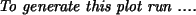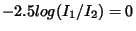Next: 5.3 Tables Up: 5.2 Images Previous: 5.2.3 Extragalactic velocity field   Contents   Index

## 5.2.4 Integrated Color Maps

A true color map can be created from a snapshot by assigning a color to the particles in the snapshot. This can be done by gridding the snapshots twice, each one assigning the particles with a different emissivity. In the example below a snapshot is assigned an artificial radial color gradient. The particles are given a linearly increasing emissivity, according to their ranking in radius. First, we must make sure the snapshot is sorted in radius properly:

```    30% snapsort in=snap.dat out=tmp1 rank=r
```

and next the snapshot is gridded twice:

```    31% snapgrid in=tmp1 out=tmp1_1 evar=m
32% snapgrid in=tmp1 out=tmp1_2 evar='m*(i+1)'
```

This means the color at the center would be, whereas at the edge the color would be. The images are best viewed when smoothed, and then divided and taken the log of. The factor 2.5 is left out here, because the scaling is arbitrary:

```    33% ccdsmooth in=tmp1_1 out=tmp1_1s gauss=0.3
34% ccdsmooth in=tmp1_2 out=tmp1_2s gauss=0.3
35% ccdmath in=tmp1_1s,tmp1_2s out=color_ccd.dat fie='log(%2/%1)'
```

........... more to come

(c) Peter Teuben